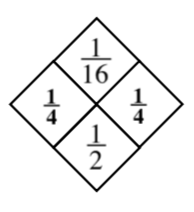Home > INT2 > Chapter 3 > Lesson 3.2.2 > Problem3-85

3-85.

Copy and complete each of the Diamond Problems below. The pattern used in the Diamond Problems is shown at right. Homework Help ✎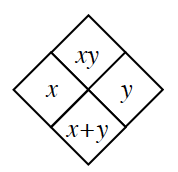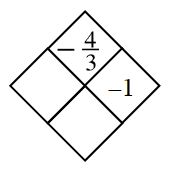Hint (a):$-1\times?=\frac{-4}{3}$More Help (a):$−1+$ (answer from above) $=?$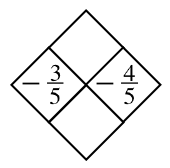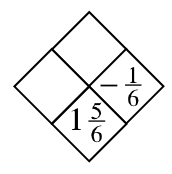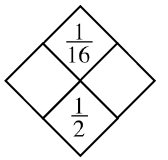1.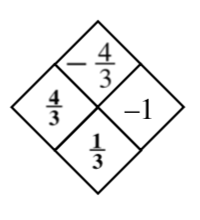1.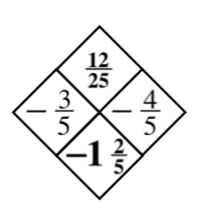1.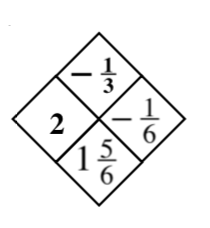1.